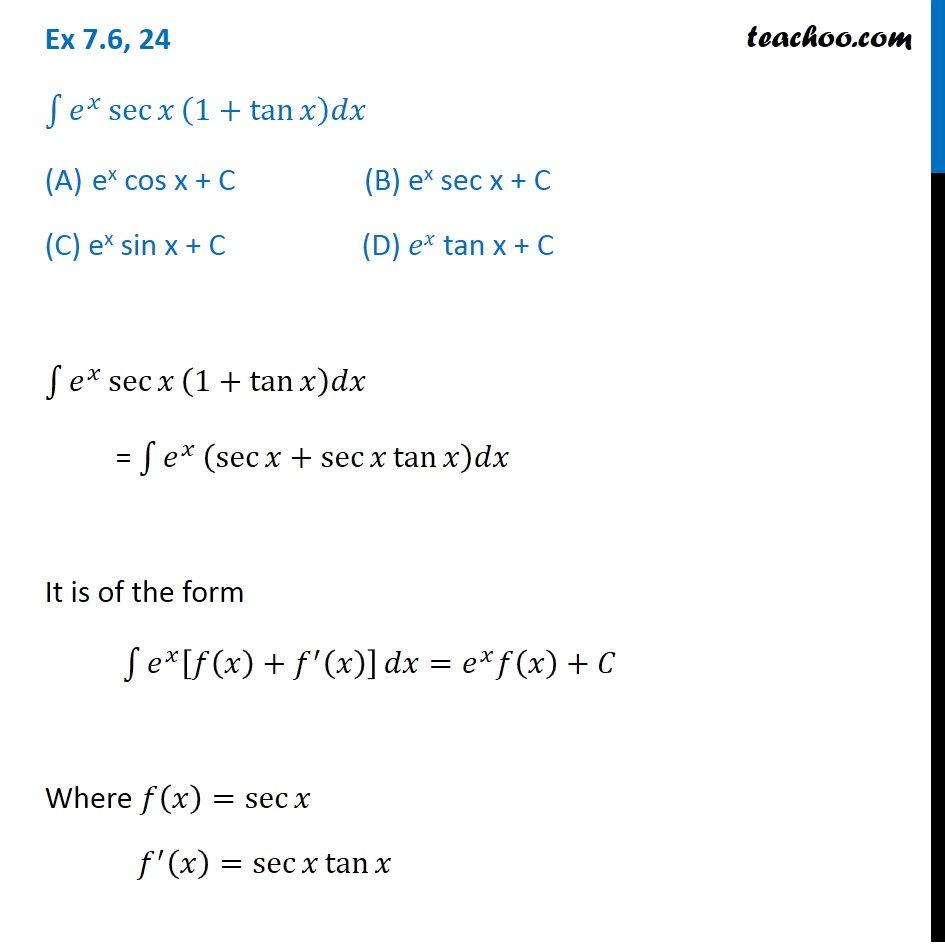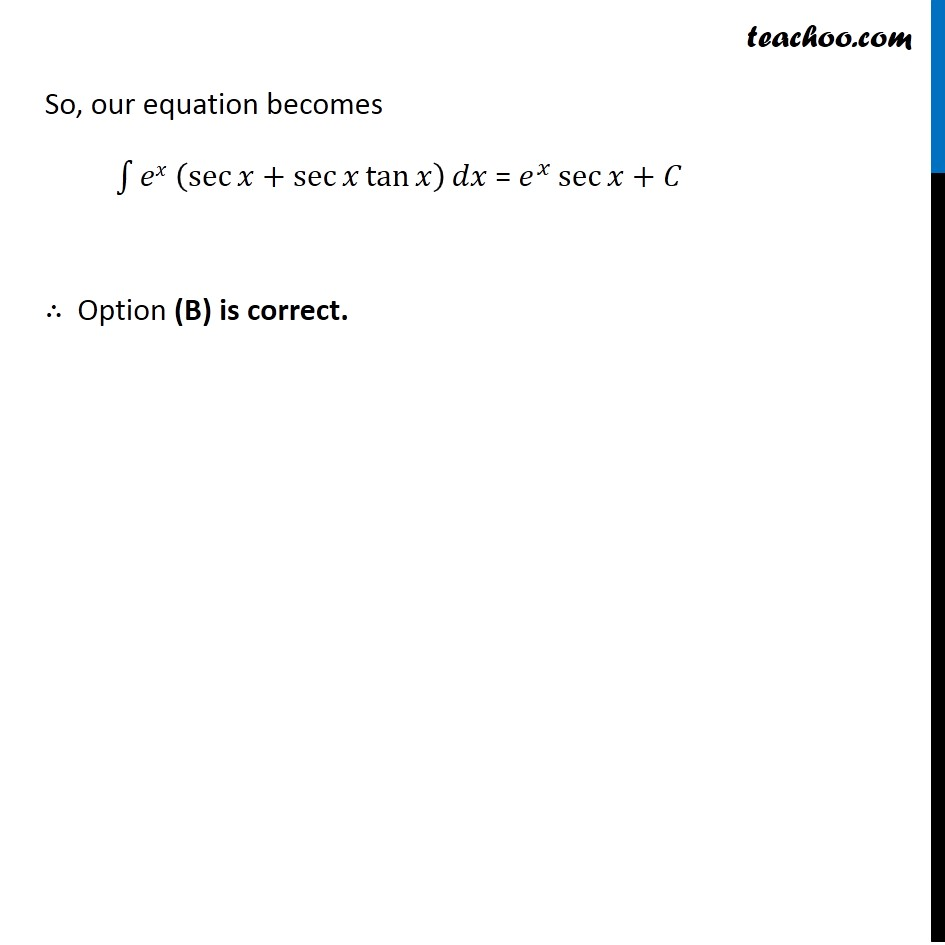Ex 7.6

Chapter 7 Class 12 Integrals (Term 2)
Serial order wise### Transcript

Ex 7.6, 24 ∫1▒𝑒^𝑥 sec⁡𝑥 (1+tan⁡𝑥 )𝑑𝑥 "ex" cos x + C (B) "ex" sec x + C (C) "ex" sin x + C (D) 𝑒𝑥 tan x + C ∫1▒𝑒^𝑥 sec⁡𝑥 (1+tan⁡𝑥 )𝑑𝑥 = ∫1▒𝑒^𝑥 (sec⁡𝑥+sec⁡𝑥 tan⁡𝑥 )𝑑𝑥 It is of the form ∫1▒〖𝑒^𝑥 [𝑓(𝑥)+𝑓^′ (𝑥)] 〗 𝑑𝑥=𝑒^𝑥 𝑓(𝑥)+𝐶 Where 𝑓(𝑥)=sec⁡𝑥 𝑓^′ (𝑥)=sec⁡𝑥 tan⁡𝑥 So, our equation becomes ∫1▒〖𝑒𝑥 (sec⁡𝑥+sec⁡𝑥 tan⁡𝑥 ) 〗 𝑑𝑥 = 𝑒^𝑥 sec⁡𝑥+𝐶 ∴ Option (B) is correct.